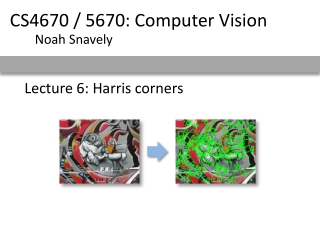DownloadDownload PresentationLecture 6: Harris corners

# Lecture 6: Harris corners

Download Presentation## Lecture 6: Harris corners

- - - - - - - - - - - - - - - - - - - - - - - - - - - E N D - - - - - - - - - - - - - - - - - - - - - - - - - - -
##### Presentation Transcript

1. CS4670 / 5670: Computer Vision Noah Snavely Lecture 6: Harris corners

3. Feature extraction: Corners and blobs

4. Local measure of feature uniqueness • How does the window change when you shift it? • Shifting the window in any direction causes a big change “corner”:significant change in all directions “flat” region:no change in all directions “edge”: no change along the edge direction Credit: S. Seitz, D. Frolova, D. Simakov

5. Harris corner detection: the math • Consider shifting the window W by (u,v) • how do the pixels in W change? • compare each pixel before and after bysumming up the squared differences (SSD) • this defines an SSD “error” E(u,v): W

6. Small motion assumption • Taylor Series expansion of I: • If the motion (u,v) is small, then first order approximation is good • Plugging this into the formula on the previous slide…

7. Harris corner detection: the math Using the small motion assumption, replace Iwith a linear approximation W (Shorthand: )

8. Corner detection: the math W • Thus, E(u,v) is locally approximated as a quadratic form

9. The second moment matrix The surface E(u,v) is locally approximated by a quadratic form. Let’s try to understand its shape.

10. E(u,v) Horizontal edge: v u

11. E(u,v) Vertical edge: v u

12. direction of the fastest change direction of the slowest change (max)-1/2 (min)-1/2 General case The shape of H tells us something about the distribution of gradients around a pixel We can visualize H as an ellipse with axis lengths determined by the eigenvalues of H and orientation determined by the eigenvectors of H max,min: eigenvalues of H Ellipse equation:

13. Quick eigenvalue/eigenvector review The eigenvectors of a matrix A are the vectors x that satisfy: The scalar  is the eigenvalue corresponding to x • The eigenvalues are found by solving: • In our case, A = H is a 2x2 matrix, so we have • The solution: Once you know , you find x by solving

14. Corner detection: the math xmin xmax • Eigenvalues and eigenvectors of H • Define shift directions with the smallest and largest change in error • xmax = direction of largest increase in E • max = amount of increase in direction xmax • xmin = direction of smallest increase in E • min = amount of increase in direction xmin

15. Corner detection: the math • How are max, xmax, min, and xmin relevant for feature detection? • What’s our feature scoring function?

16. Corner detection: the math • How are max, xmax, min, and xmin relevant for feature detection? • What’s our feature scoring function? • Want E(u,v) to be large for small shifts in all directions • the minimum of E(u,v) should be large, over all unit vectors [u v] • this minimum is given by the smaller eigenvalue (min) of H

17. Interpreting the eigenvalues Classification of image points using eigenvalues of M: 2 “Edge” 2 >> 1 “Corner”1 and 2 are large,1 ~ 2;E increases in all directions 1 and 2 are small;E is almost constant in all directions “Edge” 1 >> 2 “Flat” region 1

18. Corner detection summary • Here’s what you do • Compute the gradient at each point in the image • Create the H matrix from the entries in the gradient • Compute the eigenvalues. • Find points with large response (min> threshold) • Choose those points where min is a local maximum as features

19. Corner detection summary • Here’s what you do • Compute the gradient at each point in the image • Create the H matrix from the entries in the gradient • Compute the eigenvalues. • Find points with large response (min> threshold) • Choose those points where min is a local maximum as features

20. The Harris operator • min is a variant of the “Harris operator” for feature detection • The trace is the sum of the diagonals, i.e., trace(H) = h11 + h22 • Very similar to min but less expensive (no square root) • Called the “Harris Corner Detector” or “Harris Operator” • Lots of other detectors, this is one of the most popular

21. The Harris operator Harris operator

22. Harris detector example

23. f value (red high, blue low)

24. Threshold (f > value)

25. Find local maxima of f

26. Harris features (in red)

27. Weighting the derivatives • In practice, using a simple window W doesn’t work too well • Instead, we’ll weight each derivative value based on its distance from the center pixel

28. Questions?